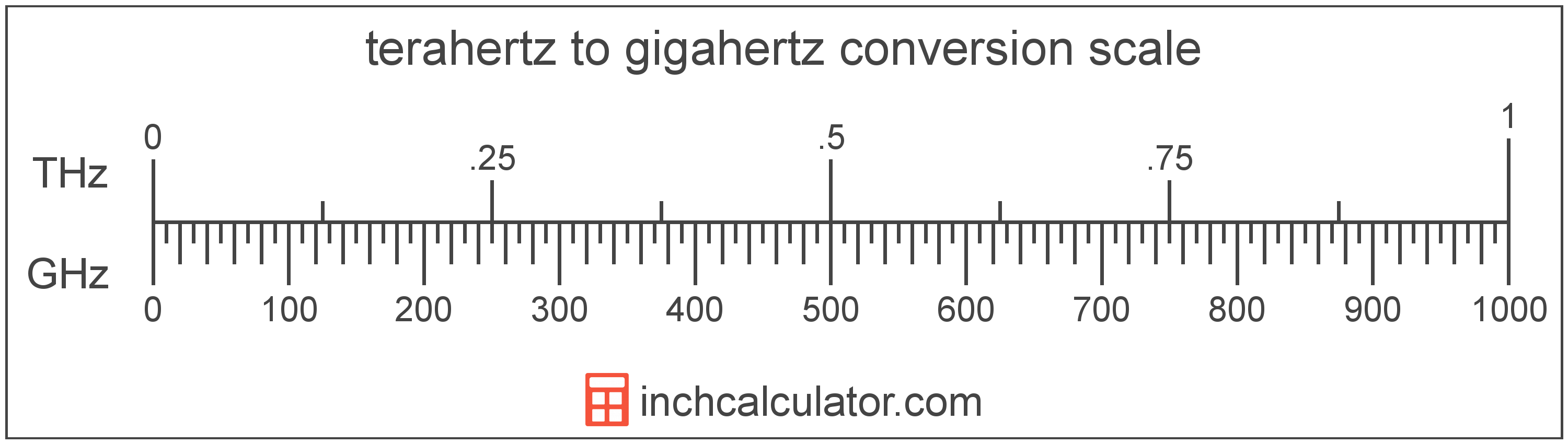# Gigahertz to Terahertz Converter

Enter the frequency in gigahertz below to get the value converted to terahertz.

Results in Terahertz:1 GHz = 0.001 THz

Do you want to convert terahertz to gigahertz?

## How to Convert Gigahertz to Terahertz

To convert a measurement in gigahertz to a measurement in terahertz, divide the frequency by the following conversion ratio: 1,000 gigahertz/terahertz.

Since one terahertz is equal to 1,000 gigahertz, you can use this simple formula to convert:

terahertz = gigahertz ÷ 1,000

The frequency in terahertz is equal to the frequency in gigahertz divided by 1,000.

For example, here's how to convert 5,000 gigahertz to terahertz using the formula above.
terahertz = (5,000 GHz ÷ 1,000) = 5 THz## What Is a Gigahertz?

Gigahertz is a measure of frequency equal to one billion cycles per second.

The gigahertz is a multiple of the hertz, which is the SI derived unit for frequency. In the metric system, "giga" is the prefix for billions, or 109. Gigahertz can be abbreviated as GHz; for example, 1 gigahertz can be written as 1 GHz.

## What Is a Terahertz?

Terahertz is a measure of frequency equal to one trillion cycles per second.

The terahertz is a multiple of the hertz, which is the SI derived unit for frequency. In the metric system, "tera" is the prefix for 1012. Terahertz can be abbreviated as THz; for example, 1 terahertz can be written as 1 THz.

## Gigahertz to Terahertz Conversion Table

Table showing various gigahertz measurements converted to terahertz.
Gigahertz Terahertz
1 GHz 0.001 THz
2 GHz 0.002 THz
3 GHz 0.003 THz
4 GHz 0.004 THz
5 GHz 0.005 THz
6 GHz 0.006 THz
7 GHz 0.007 THz
8 GHz 0.008 THz
9 GHz 0.009 THz
10 GHz 0.01 THz
20 GHz 0.02 THz
30 GHz 0.03 THz
40 GHz 0.04 THz
50 GHz 0.05 THz
60 GHz 0.06 THz
70 GHz 0.07 THz
80 GHz 0.08 THz
90 GHz 0.09 THz
100 GHz 0.1 THz
200 GHz 0.2 THz
300 GHz 0.3 THz
400 GHz 0.4 THz
500 GHz 0.5 THz
600 GHz 0.6 THz
700 GHz 0.7 THz
800 GHz 0.8 THz
900 GHz 0.9 THz
1,000 GHz 1 THz